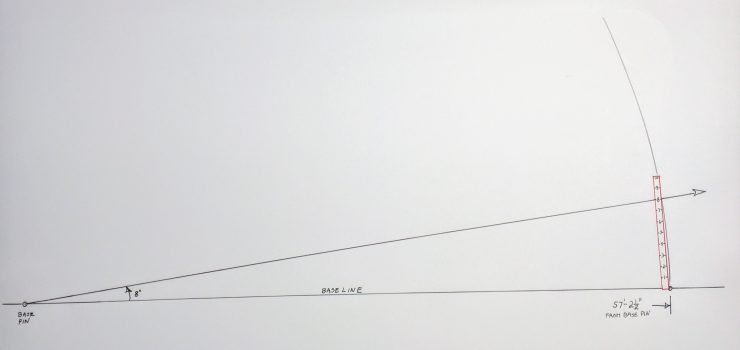Here’s an old school carpenter’s (or landscaper’s) method of laying out a line–such as a foundation form or a hedge row–to a specified angle. The tools needed are simple–primitive even: A length of rope marked at a certain distance and a ten-foot pole marked in one-ft. Increments (i.e. the once ubiquitous carpenter’s ten-foot pole). Or you can join the twentieth century and use a tape measure. Let’s jump right in and lay out an eight degree angle from a baseline. The drawing below is pretty self explanatory but I’ll explain it anyway in my hopefully not too pedantic step-by-step fashion.Step 1:  Establish the baseline (via a stretched string) and set a pin (a sharpened stick works) at the focal point to which the angle will converge.

Step 2:  Make a loop at the end of a non-stretchable rope (i.e. avoid nylon) and run it out along the baseline from the base pin. Measure out 57-feet, 2 ½-inches from the pin along the rope and make a mark with a sharpie or tie on a piece of string. Also set a pin at the baseline at that distance.

Step 3:  Now arc the rope away from the baseline in the direction you want to lay out the angle.

Step 4: Set the base of the ten-foot pole at the base line pin and orient it to the rope. When the eight-foot mark on the pole passes over the mark on the rope the angle to the baseline is (drum roll) eight degrees.

So how does this work you might ask? As my friend Joe Youcha of buildingtoteach.com explained to me:  “The answer is buried in the math we were all injected with in grammar school.” We were all told about the “transcendental number” called “pi” which when inputted into your calculator would provide you with either the circumference of a circle based on its diameter or vice versa.

Artisans of antiquity, however, had no knowledge of the decimal number pi. In fact decimal numbers in general had not been described in detail in the western world until the late 1500’s by the mathematician Simon Stevin. But artisans did have an excellent working relationship with the straightforward (non-cendental?) proportional ratio system. In the case of the relationship of the diameter of a circle with its circumference, they would just step out the diameter into seven segments and know that twenty-two of those segments would, to a high level of accuracy, give them the length of the circumference. Good enough for government work (such as the Parthenon) as they say.

Since we apparently need to work with degrees (probably because the architect speced out the angle in degrees instead of the length of a chord as they would have in antiquity) we would need to know what number of segments the diameter would be if the circumference were stepped out to 360 segments. (That number is, of course, an arbitrary but widely accepted convention since Babylonian times as a convenient way to divvy up a circle. We like it as it can be evenly divided by so many whole number divisions–though for a time Europeans were quite fond of 400 degrees.

But I digress, back to how it works: If you go to the trouble of physically stepping out along the circumference of a circle with dividers, you’ll discover that when three hundred and sixty segments do the trick, one hundred and fourteen and five twelfths of another segment will define the diameter. Of course, using al-Jabr ( given to us by the Islamic mathematicians), we can quickly solve for this result using an algebraic equation to solve for an unknown.

For this purpose we’ll use half of the diameter segments–fifty seven and three and one half twelfths–to lay out the radius length on the rope. The bottom line: We find that a radius of 57 feet, 3 ½ inches produces a circumference length of 360 feet. So for every foot we swing the arc, we produce an angle of one degree.